One of the benefits of using the SWEEP operator is that it enables you to "sweep in" columns (add effects to a model) in any order. This article shows that if you use the SWEEP operator, you can compute a SSCP matrix and use it repeatedly to estimate any linear regression model that uses those effects.

This post relates to a previous post, in which I showed that you never need to use a permutation matrix to rearrange the order of rows and columns of a matrix. As an application, I used the sum of squares and crossproducts (SSCP) matrix, which is used to estimate the regression coefficients in a least-squares regression model. Being able to permute the rows and columns of the SSCP matrix efficiently means that you can solve the linear regression problem very quickly for any ordering of the columns of the design matrix. But if you use the SWEEP operator, you do not need to permute the SSCP matrix at all!

### The SSCP matrix in PROC REG

Before discussing the SWEEP operator, let's review a little-used feature of PROC REG in SAS. The REG procedure is interactive, which means that you can interactively add or delete effects from a model as part of the model-building process. However, if you are going to explore several different models, you must use the VAR statement to specify all variables that you might conceivably want to use BEFORE the first RUN statement. Why? Because when PROC REG encounters the RUN statement it builds the SSCP matrix for the variables that you have specified. As stated in the documentation, for each subsequent model, "the procedure selects the appropriate crossproducts from the [SSCP] matrix." In other words, PROC REG re-uses that SSCP matrix for every MODEL statement.

Let's look at an example. The following call to PROC REG computes the SSCP matrix for five variables and the intercept effect. It uses that SSCP matrix to compute the parameter estimates:

```proc reg data=sashelp.cars plots=NONE; ods select ParameterEstimates; VAR MSRP EngineSize Horsepower MPG_Highway Weight; /* vars in the SSCP */ FULL: model MSRP = EngineSize Horsepower MPG_Highway Weight; /* uses the SSCP */ run;```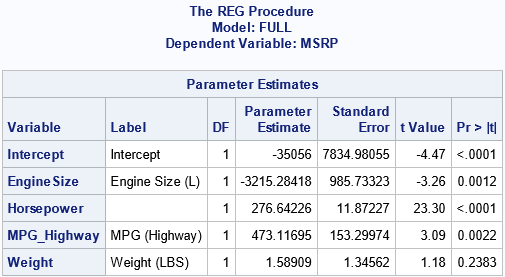Because PROC REG is an interactive procedure, the procedure does not exit when it encounters the RUN statement. It remembers the SSCP matrix until the procedure exits. If you submit additional MODEL statements, PROC REG will use the SSCP matrix to estimate the new parameters, as long as the variables were previously specified. For example, you can specify the same model, but list the variables in a different order. Or you can estimate a reduced model that contains only a subset of the variables, as follows:

``` PERMUTE: model MSRP = Horsepower Weight EngineSize MPG_Highway; run; PARTIAL: model MSRP = Weight Horsepower; run;quit;```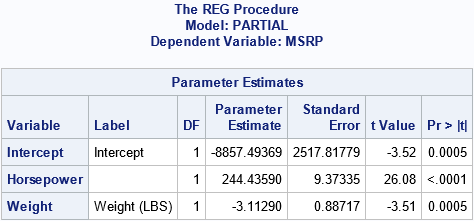The output for the "PERMUTE" model is not shown, because the estimates are the same as for the "FULL" model, but in a different order. It is important to note that PROC REG estimates the second and third models without re-reading the data. It simply re-uses the original SSCP matrix. As I have previously shown, computing the SSCP matrix is often 90% of the work of computing regression estimates, which means that the second and third models are computed very quickly.

The next section shows that how the SWEEP operator can quickly compute the parameter estimates for any model and for any order of the variables. There is no need to physically permute the rows and columns of the SSCP matrix.

### The SWEEP operator

You can use the SAS/IML language to illuminate how PROC REG can compute one SSCP matrix and re-use it for all subsequent models. The following SAS/IML statements read the data and compute the SSCP matrix. Following Goodnight (1979), the last column contains the response variable, but this is merely a convenience.

```proc iml; varNames = {'EngineSize' 'Horsepower' 'MPG_Highway' 'Weight'}; use sashelp.cars; read all var varNames into X; read all var 'MSRP' into Y; close; /* add intercept column and response variable */ X = j(nrow(X), 1, 1) || X; /* the design matrix */ k = ncol(X); /* number of effects */   varNames = 'Intercept' || varNames || 'MSRP'; XY = X || Y; SSCP = XY` * XY; /* the only SSCP that we'll need */   /* by using the sweep operator (1st row=mean, last col=param est*/ S = sweep(SSCP, 1:k); rCol = nrow(SSCP); /* the response column */ b = S[1:k, rCol]; /* parameter estimates for regression coefficients */ print b[r=varNames];```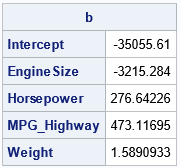The parameter estimates are the same as computed by PROC REG. They were obtained by sweeping the columns of the SSCP matrix in the order 1, 2, ..., 5. You can think of the vector v=1:k as the "identity permutation" of the rows. Then the SWEEP operator for the variables in their original order is the operation S = sweep(SSCP, v).

The SWEEP function in SAS/IML enables you to specify any vector of indices as the second argument. In this way, you can sweep the columns of the SSCP matrix in any order. For example, the "PERMUTE" model from the PROC REG example corresponds to sweeping the SSCP matrix in the order v = {1 3 5 2 4}. As the following example shows, you get the same parameter estimates because the models are the same:

```/* sweep original SSCP in the order of v */ v = {1 3 5 2 4}; pVarNames = varNames[,v]; /* effect names in permuted order */ S = sweep(SSCP, v); /* sweep the specified rows in the specified order */ b = S[v, rCol]; /* get the parameter estimates in new order */ print b[r=pVarNames];```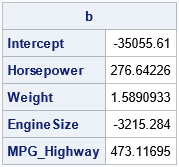As expected, the parameter estimates are the same, but the order of the parameters is different.

You can also estimate parameters for any model that uses a subset of the columns. For example, the model that has the explanatory variables Weight and Horsepower (the fifth and third effects) is computed from the SSCP matrix by sweeping the columns in the order {1 5 3}, as follows:

```/* Perform a partial sweep for the model {1 5 3}. No need to form a new design matrix or to extract a portion of the SCCP. */ v = {1 5 3}; pVarNames = varNames[,v]; /* effect names for this model */ S = sweep(SSCP, v); /* sweeps in the variables in the order of v */ b = S[v, rCol]; /* get the parameter estimates */ print b[r=pVarNames];```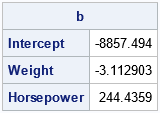The output matches the "PARTIAL" model from PROC REG.

### Summary

In a previous post, I showed an efficient way to permute the order of rows and columns of a matrix. But the current article shows that you do not need to permute the elements of an SSCP matrix if you use the SWEEP operator. The SWEEP operator enables you to specify the order in which you want to add effects to the model. From a design matrix, you can compute the SSCP matrix. (This is most of the work!) You can then quickly compute any model that uses the columns of the design matrix in any order.

This useful property of the SWEEP operator is among the many features discussed in Goodnight, J. (1979), "A Tutorial on the SWEEP Operator," The American Statistician. For other references, see the end of my previous article about the SWEEP operator.

The post More on the SWEEP operator for least-square regression models appeared first on The DO Loop.